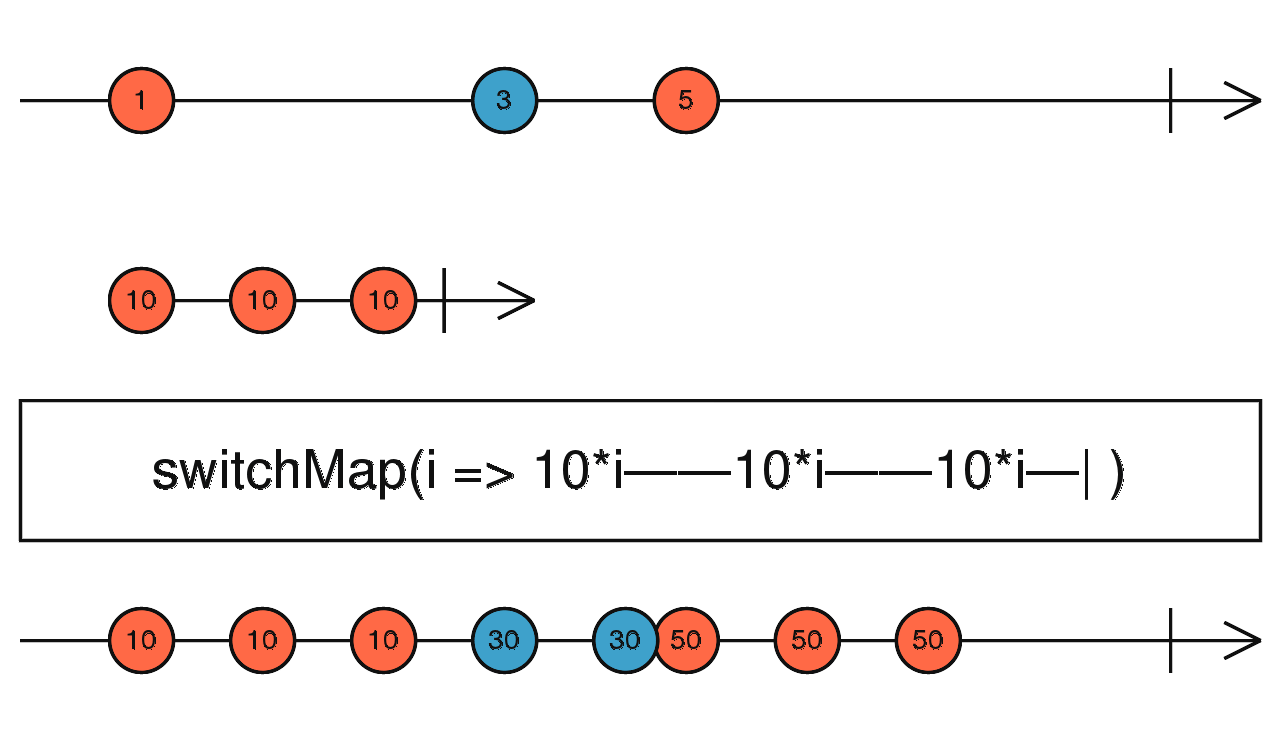/RxJS

# switchMap

`function` `stable`

Projects each source value to an Observable which is merged in the output Observable, emitting values only from the most recently projected Observable.

### `switchMap<T, R, O extends ObservableInput<any>>(project: (value: T, index: number) => O, resultSelector?: (outerValue: T, innerValue: ObservedValueOf<O>, outerIndex: number, innerIndex: number) => R): OperatorFunction<T, ObservedValueOf<O> | R>`

#### Parameters

 project A function that, when applied to an item emitted by the source Observable, returns an Observable. resultSelector Optional. Default is `undefined`. Type: `(outerValue: T, innerValue: ObservedValueOf, outerIndex: number, innerIndex: number) => R`.

#### Returns

`OperatorFunction<T, ObservedValueOf<O> | R>`: An Observable that emits the result of applying the projection function (and the optional deprecated `resultSelector`) to each item emitted by the source Observable and taking only the values from the most recently projected inner Observable.

## Description

Maps each value to an Observable, then flattens all of these inner Observables.Returns an Observable that emits items based on applying a function that you supply to each item emitted by the source Observable, where that function returns an (so-called "inner") Observable. Each time it observes one of these inner Observables, the output Observable begins emitting the items emitted by that inner Observable. When a new inner Observable is emitted, `switchMap` stops emitting items from the earlier-emitted inner Observable and begins emitting items from the new one. It continues to behave like this for subsequent inner Observables.

## Example

Generate new Observable according to source Observable values

```import { of } from 'rxjs';
import { switchMap } from 'rxjs/operators';

const switched = of(1, 2, 3).pipe(switchMap((x: number) => of(x, x ** 2, x ** 3)));
switched.subscribe(x => console.log(x));
// outputs
// 1
// 1
// 1
// 2
// 4
// 8
// ... and so on```

Rerun an interval Observable on every click event

```import { fromEvent, interval } from 'rxjs';
import { switchMap } from 'rxjs/operators';

const clicks = fromEvent(document, 'click');
const result = clicks.pipe(switchMap((ev) => interval(1000)));
result.subscribe(x => console.log(x));```

### `switchMap(project: (value: T, index: number) => O): OperatorFunction<T, ObservedValueOf<O>>`

#### Parameters

 project Type: `(value: T, index: number) => O`.

#### Returns

`OperatorFunction<T, ObservedValueOf<O>>`

### `switchMap(project: (value: T, index: number) => O, resultSelector: undefined): OperatorFunction<T, ObservedValueOf<O>>`

#### Parameters

 project Type: `(value: T, index: number) => O`. resultSelector Type: `undefined`.

#### Returns

`OperatorFunction<T, ObservedValueOf<O>>`

### `switchMap(project: (value: T, index: number) => O, resultSelector: (outerValue: T, innerValue: ObservedValueOf<O>, outerIndex: number, innerIndex: number) => R): OperatorFunction<T, R>`

#### Parameters

 project Type: `(value: T, index: number) => O`. resultSelector Type: `(outerValue: T, innerValue: ObservedValueOf, outerIndex: number, innerIndex: number) => R`.

#### Returns

`OperatorFunction<T, R>`